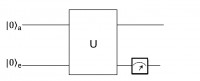June 2011
Mon Tue Wed Thu Fri Sat Sun
« May   Jul »
12345
6789101112
13141516171819
20212223242526
27282930

## Time-Reversal Symmetry in Non-Hermitian Systems

Masatoshi Sato, Kazuki Hasebe, Kenta Esaki, Mahito Kohmoto

For ordinary hermitian Hamiltonians, the states show the Kramers degeneracy when the system has a half-odd-integer spin and the time reversal operator obeys $$\Theta^2=-1$$, but no such a degeneracy exists when $$\Theta^2=+1$$. Here we point out that for non-hermitian systems, there exists a degeneracy similar to Kramers even when $$\Theta^2=+1$$. It is found that the new degeneracy follows from the mathematical structure of split-quaternion, instead of quaternion from which the Kramers degeneracy follows in the usual hermitian cases. Furthermore, we also show that particle/hole symmetry gives rise to a pair of states with opposite energies on the basis of the split quaternion in a class of non-hermitian Hamiltonians. As concrete examples, we examine in detail NxN Hamiltonians with N=2 and 4 which are non-hermitian generalizations of spin 1/2 Hamiltonian and quadrupole Hamiltonian of spin 3/2, respectively.

http://arxiv.org/abs/1106.1806
Statistical Mechanics (cond-mat.stat-mech); Mathematical Physics (math-ph); Quantum Physics (quant-ph)

## Reply on Comments on “Observation of a Fast Evolution in a Parity-time-symmetric System”(ArXiv:1106.1550)

Chao Zheng, Liang Hao, Gui Lu Long

Masillo  commented on our manuscript  “Observation of a Fast Evolution in a Parity-time-symmetric System”, pointing out a contradiction of our work with Ref.. In this reply, we pointed out there is no disagreement between Masillo’s comment and our work in Ref. . The efficiency cost pointed out in Ref.\cite{masillo} exists, namely to obtain the PT-symmetric hamiltonian evolution, one has to make a measurement on the auxiliary qubit and the auxiliary qubit is at state $$\left|0\right \rangle$$ only probabilistically. This is reflected in the amplitude of the spectrum in the NMR quantum simulation. As a result, we made a small modification in a new version of the Ref. , and Fig. 2 of Ref. has been replaced by spectra of two different $$\alpha$$’s in order to illustrate this fact.

http://arxiv.org/abs/1106.1848
Quantum Physics (quant-ph)

## Comments on “Observation of Fast Evolution in Parity-Time-Symmetric System”

Fabio MasilloIn the paper “Observation of Fast Evolution in Parity-Time-Symmetric System” the authors propose a physical apparatus for the realization of a faster than Hermitian evolution. This last appears in contrast with the conclusions obtained in our paper “Some Remarks on Quantum Brachistochrone”. We will clarify this apparent contradiction and some problematic aspects of the treatment in .

http://arxiv.org/abs/1106.1550
Quantum Physics (quant-ph)